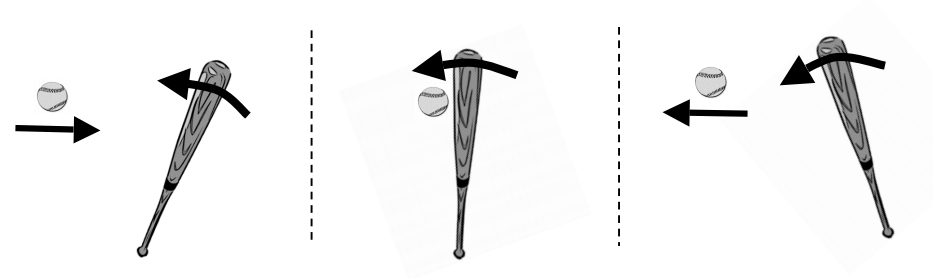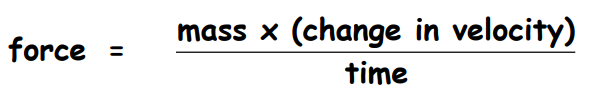Subject:
Science

Topics: ForceMotion
Concepts:

• Force (explicit)
• Acceleration (implicit)
• Momentum (implicit)

Note: Note: “Explicit” refers to concepts, which are named and discussed in the course of the lesson; “implicit” refers to concepts which students experience in the course of the lesson but which are not named and discussed.
Knowledge and Skills:

• Can analyze real world situations involving forces in terms of Newton’s Laws of Motion.

Lesson:

Procedure:

Explain to students that you are going to show them a short video which presents an example of the relationship between force and motion, and that you want them to describe how that relationship is shown when the video is over. Then show the Futures Channel Movie, “Testing Bats.”

When the movie is over, ask students to write one sentence that describes how the movie showed an example of the relationship between force and motion (alternatively, you may wish to discuss that as a class.) Use the ensuing discussion as an opportunity to review (or introduce) Newton’s first and second laws of motion.

In your discussion, ask students to decide whether how the amount of force that is required to change the velocity of the baseball by a certain amount is related to a) the amount of change in velocity, b) the mass of the object and c) the duration of time that the force is applied.

Distribute the handout to students working in teams of two or three, and ask them to read it and answer the questions given. As they do so, circulate and ask questions to ensure that students understand the equation relating force, mass, change of velocity and time.

Here are some specific questions that you may wish to ask:

1) Why would it take more force to change the velocity of the baseball as described if the duration of the impact (the time) were shorter?

2) Why would it take more force to change the velocity of the baseball as described if the mass of the ball were greater?

3) Why is the change in velocity of the baseball computed as 120 meters per second? Question #3 on the handout provides an opportunity to review (or introduce) Newton’s Third Law of Motion.

#1: 18,000 Newtons

#2: 4,050 pounds (about two tons)

#3: 4,050 pounds

### Two-Ton Baseball

When a bat hits a baseball, it changes the velocity of the ball.Any time the velocity of an object changes, we know that force has been applied.

The amount of force applied can be found from this equation:In this equation, the “time” is how long the force was applied to the object.

When a bat hits a baseball, they are in contact for around 1 millisecond (.001 seconds), so that is the time.

Typically, a baseball can be thrown toward the batter at a velocity of about 50 meters per second, and when it’s hit, it moves away from the bat at a velocity of around 70 meters per second. The total change in velocity, then, is about 120 meters per second.

The mass of a baseball is about 0.15 kilograms.

1) When you are using units of kilograms, meters, and seconds in the above equation, the force unit will be “newtons.” How many newtons of force are applied by the baseball to the bat?

2) You can convert newtons to pounds by multiplying by 0.225. How many pounds of force are applied to the baseball?

3) How many pounds of force are applied by the baseball to the bat? How do you know?